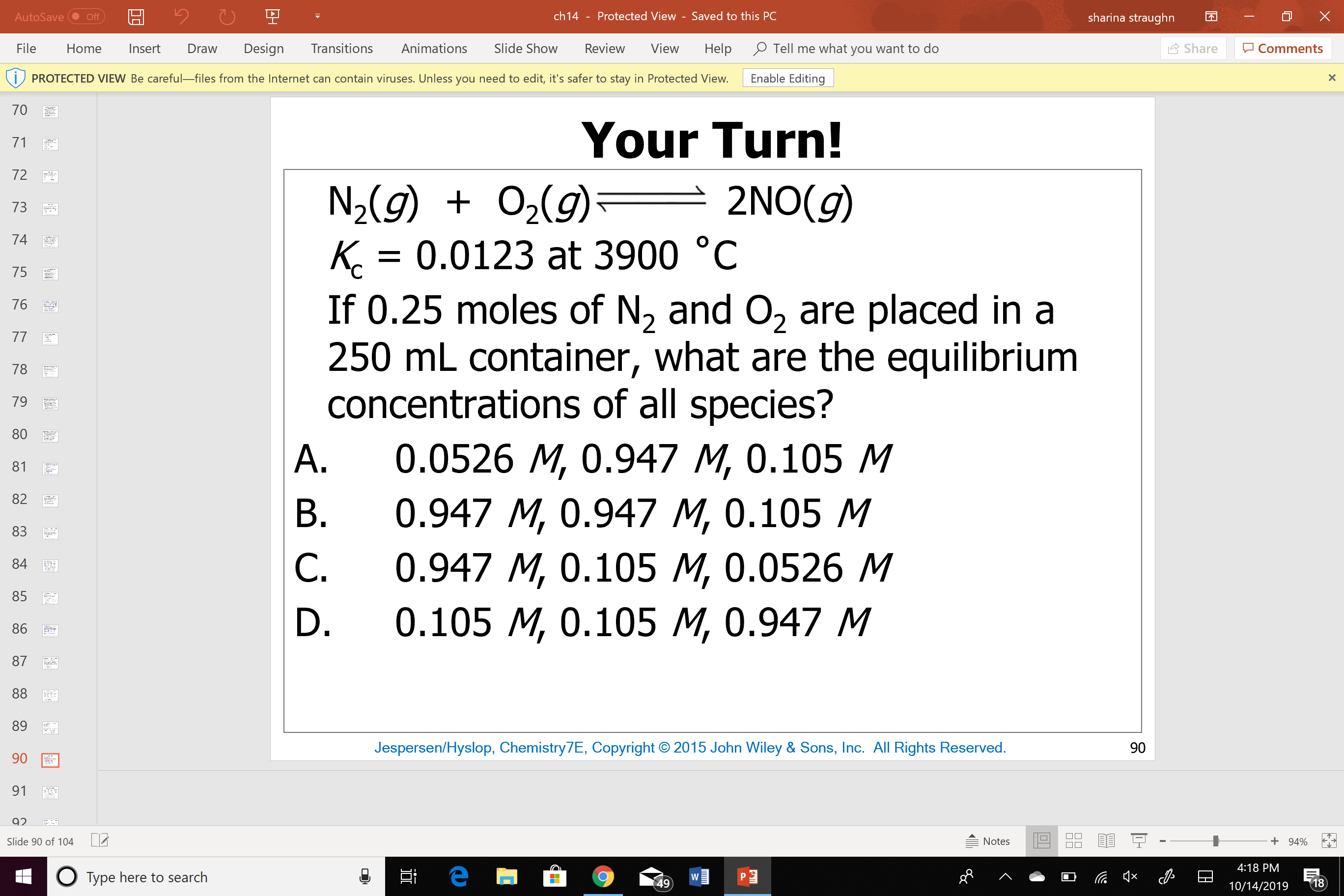# N2 (g)+ O2(g) = NO(g) Kc = 0.0123 at 3900 deg C If 0.25 moles of N2 and O2 are placed in a 250 mL container what are the equilibrium concentrations of all species. How do I solve this problem using the I.C.E. table? A. 0.0526M, 0.947 M, 0.105 M B. 0.947 M, 0.947 M, 0.105 M C.0.947 M, 0.105 M, 0.0526M, D. 0.105 M, 0.105 M, 0.947 M